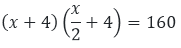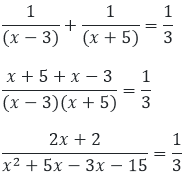×#### Thank you for registering.

One of our academic counsellors will contact you within 1 working day.

Click to Chat

1800-1023-196

+91-120-4616500

CART 0

• 0

MY CART (5)

Use Coupon: CART20 and get 20% off on all online Study Material

ITEM
DETAILS
MRP
DISCOUNT
FINAL PRICE
Total Price: Rs.

There are no items in this cart.
Continue Shopping• Complete JEE Main/Advanced Course and Test Series
• OFFERED PRICE: Rs. 15,900
• View Details

```Chapter 8: Quadratic Equations Exercise – 8.9

Question: 1

Ashu is x years old while his mother Mrs. Veena is x2 years old. Five years hence Mrs. Veena will be three times old as Ashu. Find their present ages.

Solution:

Given that Ashu's present age is x years and his mother Mrs. Veena is x2 years

Then, acc. to question, Five years later, Ashu is (x + 5) years

And his mother Mrs. Veena is (x2 + 5) years

So, x2 + 5 = 3(x + 5)

x2 + 5 = 3x + 15 x2 + 5 - 3x - 15 = 0

x2 - 5x + 2x + 10 = 0

x(x - 5) + 2(x - 5) = 0

(x - 5)(x + 2) = 0

x = 5  or x = - 2 Since, the age can never be negative

Therefore, ashu's present age is 5 years and his mother's age is 25 years.

Question: 2

The sum of the ages of a man and his son is 45 years. Five years ago, the product of their ages was four times the man's age at the time. Find their present ages.

Solution:

Let the present age of the man be x years

Then, present age of his son is = (45 - x) years

Five years ago, man's age = (x - 5) years

And his son's age = (45 - x - 5) = (40 - x) years

Then, acc.  To question, (x - 5)(40 - x) = 4(x - 5)

40x - x2 + 5x - 200

= 4x - 20 -X2 + 45x - 200

= 4x - 20 -x2 + 45x - 200 - 4x + 20

= 0 - x2 + 41x - 180 = 0

x2 - 36x - 5x + 180 = 0

x(x - 36) - 5(x - 36) = 0

(x - 36)(x - 5) = 0

x = 36  or  x = 5 But, the father's age can never be 5 years

Therefore, when x = 36, 45 - x = 45 - 36 = 9

Hence, man's present age is 36 years and his son's age is 9 years.

Question: 3

The product of Shikha's age five years ago and her age 8 years later is 30, her age at both times being given in years. Find her present age.

Solution:

Let the present age of shikha be x years

Then, 8 years later, age of her = (x + 8) years

Five years ago, her age = (x - 5) years

Then, acc. To question, (x - 5)(x +8) = 30

x2 + 8x - 5x - 40 = 30

x2 + 3x - 40 - 30 = 0

x2 + 3x - 70 = 0

x(x - 7) + 10(x - 7) = 0

(x - 7)(x + 10) = 0

x = 7 or x = -10

Since, the age can never be negative. Hence, the present age of shikha is = 7 years.

Question: 4

The product of Ramu's age (in years) five years ago and his age (in years) nine years later is 15. Determine Ramu's present age.

Solution:

Let the present age of ramu be x years

Then, 9 years later, age of her = (x + 9) years

Five years ago, her age = (x - 9) years

Then, acc. to question, (x - 5)(x + 5) = 15

x2 + 9x - 5x - 45 = 15

x2 + 4x - 45 - 15 = 0

x2 + 4x - 60 = 0

x2 - 6x + 10x - 60 = 0

x(x - 6) + 10(x - 6) = 0

(x - 6)(x + 10) = 0

x = 6  or  x = - 10 Since, the age can be never be negative

Therefore, the present age of ramu is = 6 years

Question: 5

Is the following situation possible? If so, determine their present ages. The sum of the ages of two friends is 20 years. Four years ago, the product of their ages in years was 48.

Solution:

Let the present age of two friends be x years and (20 - x) years respectively

Then, 4 years later, the age of two friends will be (x - 4) years and (20 - x - 4) years

Then, acc. To the question (x - 4)(20 - x - 4) = 48

(x - 4)(16 - x) = 48

16x - x2 - 64 + 4x = 48 - x2 + 20x - 64 - 48 = 0

x2 - 20x + 112 = 0

Let the discriminant of the above quadratic eqn.

D = b2 - 4ac

Here, a = 1, b = -20, c = 112 D = (-20)2 - (4 × 1 × 112) = 400 - 448 = - 48

Since, D < 0

The above question does not have real roots. Hence, the given situation is not possible.

Question: 6

A girl is twice as old as her sister. Four years hence, the product of their ages (in years) will be 160. Find their present ages.

Solution:

Let the present age of girl be x years then, age of her sister (x/2) years

Then, 4 years later, age of girl = (x + 4) years and her sister's age be (x/2 + 4) years

Then, acc. to the question,(x + 4) (x + 8) = 160x2

x2 + 8x + 4x + 32 = 320

x2 + 12x - 288 = 0

x2 - 12x + 24x - 288 = 0

x(x - 12) + 24(x - 12) = 0

(x - 12)(x + 24) = 0

x = 12  or x = - 24 Since, the can never be negative,

Therefore, the present age of the girl is = 12 years.

And her sister's age will be, x/2 = 12/2 = 6 years.

Question: 7

The sum of the reciprocals of Rehman's ages (in years) 3 years ago and 5 years from now is 1/3. Find his present age.

Solution:

Let the present age of Rehman be x years

Then, 8 years late, age of her = (x + 5) years

Five years ago, her age = (x - 3) years Acc. To question,x2 + 2x - 15 = 6x + 6

x2 + 2x - 15 - 6x - 6 = 0

x2 - 4x - 21 = 0

x2 - 7x + 3x - 21 = 0

x(x - 7) + 3(x - 7) = 0

(x - 7)(x + 3) = 0

x = 7 or x = - 3

Since, the age can never be negative

Therefore, the present age of Rehman be = 7 years.
```### Course Features

• 728 Video Lectures
• Revision Notes
• Previous Year Papers
• Mind Map
• Study Planner
• NCERT Solutions
• Discussion Forum
• Test paper with Video Solution